# Problem 58244. Easy Sequences 113: Almost Golden Integer Rectangles 2

A golden rectangle is a rectangle whose side lengths are in the golden ratio,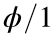, where: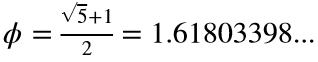.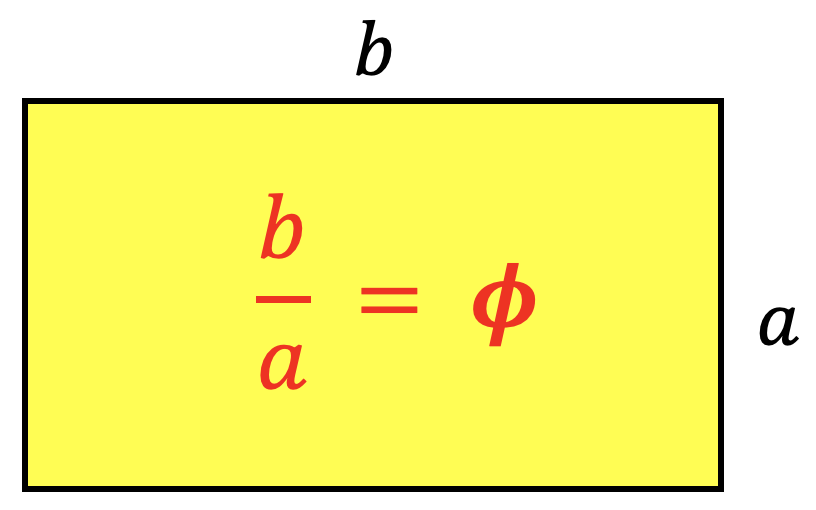The ancient Greeks consider the golden rectangle as the most aesthetically pleasing rectangular shape. Unfortunately for the ancients this ratio cannot be achieved if the sides are rational numbers let alone integers.
We define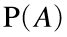as a function that calculates the perimeter of the rectangle with integer sides with area equal to A, and in which the side ratio is as close as possible to the golden ratio, that is the absolute value,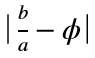, is minimized.
For example, if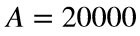, the correct dimensions should be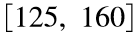, since the ratio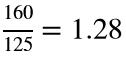is the closest we can get to ϕ for rectangle with area of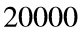(see below code). Therefore,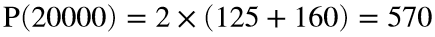.
>> A = 20000; phi = (sqrt(5)+1)/2; a = Inf;
>> for i = 1:A
if mod(A,i) == 0
j = A / i;
r = max(j/i,i/j);
if abs(r-phi) < a
ds = [i j];
a = abs(r-phi);
end
end
end
>> ds
ds =
125 160
In this problem, we are asked to evaluate the following summation:that is, the sum of perimeter of all almost golden integer rectangles with areas from 1 to A.
For example, for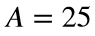, summation output should be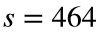: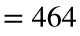### Solution Stats

100.0% Correct | 0.0% Incorrect
Last Solution submitted on Sep 17, 2023

### Community Treasure Hunt

Find the treasures in MATLAB Central and discover how the community can help you!

Start Hunting!